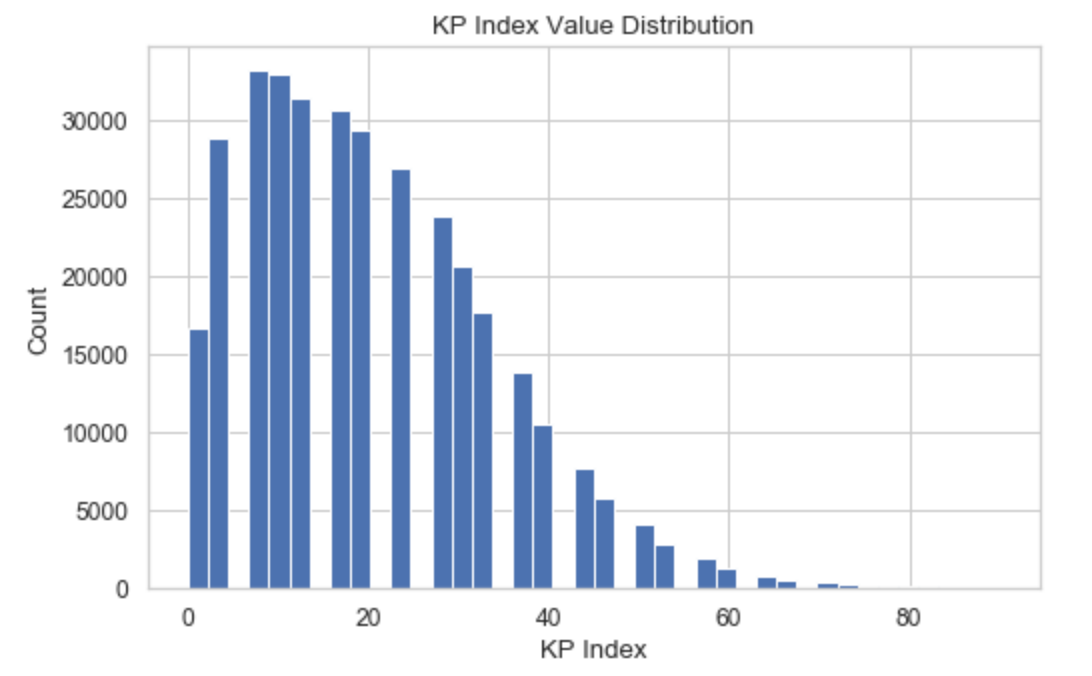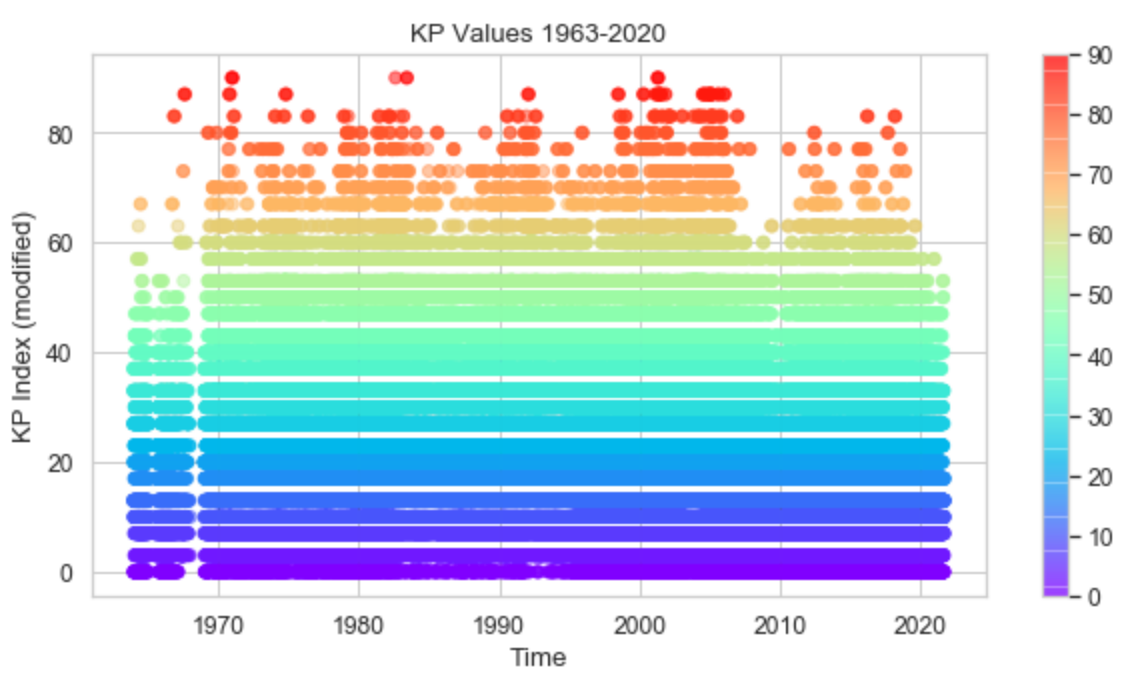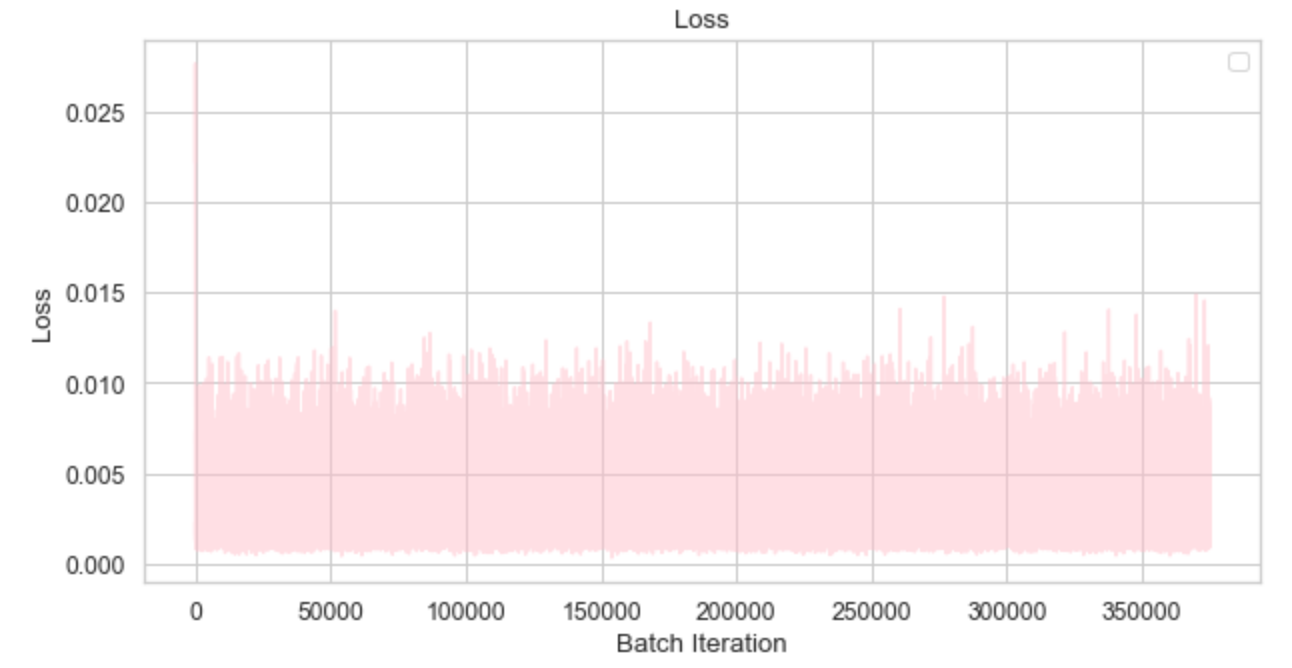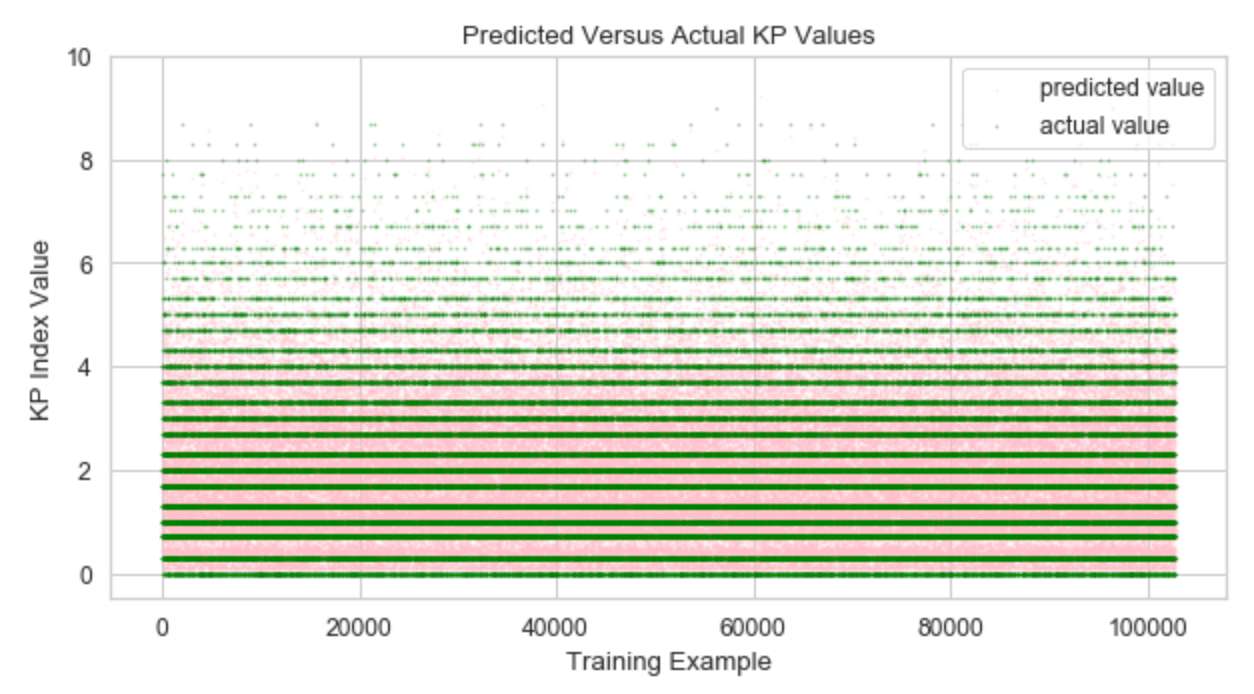Introduction

Hey hi hello! My name is Sharvani Jha, I’m a fourth year computer science major at UCLA, and I think space, machine learning, and using machine learning to further scientific knowledge or enrich experiences is a fun way to spend one’s time.

This past fall, I took a class called AOS 111: Introduction to Machine Learning for Physical Sciences with Professor Jacob Bortnik. Our final project for this class is to apply machine learning to a real dataset.

The project I chose to take on was to predict KP indices with a recurrent neural network without multiple time-lagged inputs, based on the work presented in this paper about Kp forecasting with a recurrent neural network.

I’m going to break down my explanation of this project into 3 parts:

1. High level overview — what are we actually trying to achive? (Featuring a little space weather knowledge.)

2. Everything except the machine learning: getting the data, visualizing it, preprocessing it.

3. The machine learning — the model I picked, and what my results look like.

I’ll top it all off with a conclusion, my (lengthy) list of ideas for future work + exploration, and final thoughts on how the process went and what I’ve gotten out of doing this project beyond just having a submission for my final project!

High Level Overview: Understanding the Problem

To restate again, my project’s goal was to predict KP Indices with a recurrent neural network without multiple time-Lagged inputs.

For the sake of this section, we’re going to focus on the phrase “predicting KP indices” and why that’s a problem worth exploring.

The sun is constantly producing solar wind, a stream of high-energy and charged particles that is constantly flowing outwards from the Sun and through the solar system at exceptionally high speeds.

The Planetary K-index, often called KP, characterizes the magnitude of geomagnetic storms (which are a product of our magnetosphere interacting with the solar wind). Thus, it should be possible to predict what a future KP index is based on the solar wind parameters observed close to Earth shortly before. While current models do exist for this (for example, the Wing Kp model is a recurrent neural network currently used by NASA to prepare for times of high solar activity) there is still a need for a reliable model that can forecast decently into the future to allow for better preparation during times of high solar activity.

So why use a recurrent neural network? This is actually a model that does very well when dealing with data that is composed of time series data, defined as a collection of observations obtained through repeated measurements over time. Because the previous state of the solar wind can inform us of its future states, it thus makes sense to explore this model as the ideal method to solve this problem. Thus, we will feed in the solar wind parameters as well as KP index at a certain time to help the model predict future KP values.

This problem has also been decently solved through the use of simple neural networks in the past. However, recurrent neural networks allow one to better harness and understand underlying relationships between sequential data and will most likely result in more optimal results for solving this problem (according to my current understanding of the domain).

Everything Except the Machine Learning: Preparing the Data For Training and Testing

As the paper I referenced did, I chose to use the OMNI dataset — this is an ideal dataset for this problem because:

1. There is a lot of data to use — there are many hourly measurements between 1963 to now that are available in this dataset, thus helping at least partially resolve the issue of not having enough data to train one’s model on.

2. As for guaranteeing uniformity of the data, a lot of work has been done to ensure that the values are all correct relative to each other — for instance, all the data can be assumed to have been taken at Earth’s L1 Lagrange point.

As for actually downloading the data to create the .txt file I read from to do my project, I used the `requests` and `logging` libraries to download the data from the OMNI dataset webpage.

After downloading the data, I used `seaborn` and `matplotlib` to visualize it. I plotted two important things to better guide my understanding of the situation:

1. What is the distribution of KP values across all of this data?

This allowed me to notice which KP indices were more common in the dataset. As expected, there are a larger quantity of lower values (which correspond to nominal space weather conditions, and thus lower levels of activity).kp index value distribution histogram chart made with seaborn. most values between 0 and 30, significantly fewer with values that are 35+.

2. What do the KP values look like over time?

This allowed me to see when KP values were higher versus lower over time (to a limited extent, though; there’s a lot to be done to better represent this data through plotting.)kp values from 1963–2020. the points are color-coded by their kp value. there are many more low kp values as opposed to high kp values.

Immediately, there was a concern: KP values are only supposed to be between 0–9, but in the data presented above they seem to go between 0 to 90. However, this turned out to just be a specific “quirk” of how OMNI presents its KP data — according to their descriptive text file that explains the various features in the dataset, “3+ = 33, 6- = 57, 4 = 40, etc.” — it’s just a different way to express values within the KP scale.

Next came the preprocessing.

There were a decent number of rows that had various “NaN” values — however, instead of marking NaN values with a standardized denotation, it uses varying combinations of 9’s and 0’s to create numbers that mean NaN, and these differ per feature. So I brute-force wrote down every single NaN number, set all those values to NaN, and then dropped all rows that had any NaN values.

I now had to decide what features to keep. For this, I referenced the paper I linked earlier — they conducted a parametric study to determine which parameters improved performance the most, and thus I used the same ones they did: the z-component of the magnetic field strength, the standard deviation of the absolute value of the magnetic field strength, the absolute value of the magnetic field strength, the proton number density, and the proton speed.

This means I was just left with the aforementioned features and KP values for each example.

I had to then modify my feature and target values such that feature values x(t) and y(t) would correspond to the target value of y(t+1).

Finally, I had to normalize my data — I used scikit-learn to automatically normalize all my values (both my feature and target values) to be between 0 and 1. This is to ensure that largely varying scales do not give the model an incorrect sense of which values are more important versus less important in determining the proper final output value.

I now have data that is on the same scale, and is ready to be trained and tested!

The Machine Learning: The Models, Training + Testing, and Thoughts on Results

This was it! The final (and longest) step: training the model, testing its performance, and interpreting its results to guide future work.

I primarily ran one model that worked, consisting of four main stages. The code is below for reference.

The first is the hidden layer, which takes in the inputs x(t) and y(t). In other words, this is the layer that takes in the current feature and target values at time t. The choice of inputs is determined by the coder. I chose the parameters due to the sequential nature of the data, as discussed earlier.

Applied next is an activation layer, which helps the model further understand complex relationships. The coder chooses the activation function. I chose ReLU due to its relatively high success as an activation function across various fields, but there is a lot of future work that could go into investigating which activation functions result in better model performance.

Next is the output layer, which takes the various inputs the hidden layer outputs and converts it into one output layer (which then has another activation function applied to it).

Looking at the data flow described above, one starts to get a clearer sense of how this ties back to the original goal of predicting KP values from solar wind data. The solar wind parameters and KP index at a certain time both provide important insight into what the future KP index values will be — thus, this model allows for us to calculate future KP index values based on previously known solar wind information as well as the previous KP index value itself.

The first thing one should notice is this isn’t exactly the recurrent neural network architecture described in the paper I’m referencing (for contrast, there is a picture of the architecture used by the individuals in the paper). Instead of having separate weights for x(t) and y(t) when trying to predict y(t+1), I had them both in one block of inputs. That being said, I still did include the single time-lag element by using x(t) and y(t) as inputs when trying to predict y(t+1). My future work (a.k.a. the jupyter notebook models I have running right now out of curiosity) attempt to set up the RNN as described in the paper in PyTorch, and this work defines the important next steps for this project to take.

I wrote my machine learning model (and thus handled all training and testing) in PyTorch — it’s a high-level framework that still provides relatively optimized performance, and thus was a good fit for my slightly seasoned but still-improving development purposes.

The specific model setup that ended up giving me the lowest (and thus best) testing loss value was the one shown earlier. Below is the loss function from its training, with a batch size of 64 and 100 epochs.Loss function for the best model trained. Loss sharply decreases well before 50000 iterations, and hovers around 0.01 from then onward.

Below is a figure of the actual versus predicted KP values. The predicted values are pink, and the actual values are green.A scatterplot of actual KP values versus predicted KP values. Actual values are green, predicted values are pink.

One thing that you may notice is that the actual KP values have discrete values (marked by the solid green lines that seem to appear), whereas the predicted values are on a much more continuous scale — this is because I did not round the predicted KP value to the closest valid discrete KP value. This is an area for future work, but I wanted to first understand the model’s initial performance without performing this rounding.

So how did the model do? Not too bad, actually — it has 0.2569 error, which could be worse. For tau = 1, the paper cites its average prediction error as 0.424, which is higher. While there is not a direct comparison between these two error values (since the paper’s model does round to the closest legitimate KP value and my error does not), the fact that my model’s error is relatively low (which I define as lower than 1.0) is a good indication of its performance and ensures that future work to further improve the model’s performance will be worth exploring.

However, there’s a LOT of future work that needs to be done. A couple points (especially those of interest to me) are listed below:

1. Expand on the parametric study described in the paper to determine whether there are any additional parameters it would be worth trying to train the models with.
2. Feed in and have separate sets of weights for x(t) and y(t).
3. Explore whether there a way to fill in certain NaN values with mean values such that those rows can still be used and we have more training data.
4. Try using the actual nn.RNN() architecture and compare this to the current implementation.
5. Round the KP prediction value to the nearest valid one during training and testing.
6. Perform comprehensive exploratory data analysis to gain a higher initial level of understanding of the various parameters in the data and how they relate to each other (especially the relationship between the KP index and other values).

Overall, this project was a solid initial exploration of

1. Using PyTorch to train and test a neural network for the first time
2. Using Seaborn to perform basic data visualization
3. An exploration of the setup and training + testing process for sequential data.

But most importantly, this project made me all the more interested in improving this model in my free time. Machine learning has a lot of potential, and learning how to harness it to explore my specific interests is something I’m excited to explore in the months to come.